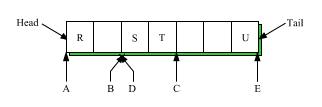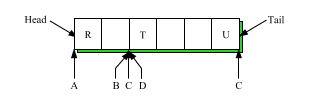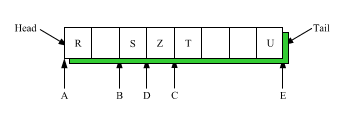DOC HOME SITE MAP MAN PAGES GNU INFO SEARCH PRINT BOOK
A List Class Library for C++ - List(C++)

# Operations on List iterators

List iterators can be assigned to, passed as arguments to functions, and returned from functions. Although there can be more than one List iterator pointing to a given List, there will be no interference between operations. However, after a List has been deleted (for example, by exiting the block in which it was declared), List iterators that pointed to it should no longer be used.

The rest of this section describes the various operations on List iterators including the linked list operations. For many linked list operations, there is an operation which is its "mirror image", whose name is the same except that "next" is replaced with "prev" and vice versa. These pairs of functions:

```   `find_next()` and `find_prev()`,
`next()` and `prev()`,
`step_next()` and `step_prev()`,
`peek_next()` and `peek_prev()`,
`remove_next()` and `remove_prev()`,
`insert_next()` and `insert_prev()`,
`replace_next()` and `replace_prev()`,
```

are symmetric in behavior with respect to direction in the List. Therefore, the semantics of an operation should always imply the semantics of its mirror image.

## Assignment

Assignment for List iterators is done with the `=` operator. The statement `Li = Li1;` changes `Li` to point to the List that `Li1` points to and its position is set to that of `Li1`. The return value of an assignment expression is the object assigned, so `Li1 = Li2 = Li3;` works as expected.

## Comparisons

The `==` and `!=` operators are used for comparisons of List iterators. `Li1 == Li2` is TRUE if `Li1` and `Li2` point to the same List and have the same position within the List. `Li1 != Li2` is TRUE otherwise.

## Checking Current Position

If `Li` is a Listiter, the statement `Li.position()` will return to the user the current position in the List. The user can find out explicitly whether the current position is at the head (or the end) of the List with the statement `Li.at_head()` (or `Li.at_end()`). `at_head()` and `at_end()` return TRUE or FALSE. In the sample diagram, `Li.position()` would return 0, `Li.at_head()` returns TRUE and `Li.at_end()` returns FALSE.

## Changing Current Position

There are six functions which change the iterator position in a List based on certain criteria.

`Li.reset(i)` sets the current position in `L` to `i`. If the argument is missing, it is assumed to be 0. `Li.end_reset(i)` sets the current position in `L` to be `i` positions from the end. If the argument is missing, it is assumed to be 0. Figure 3 shows the effect of `reset()` on a List.

```              L = ( a b c d )
^
Li.reset()      ==>   L = ( a b c d )
^
Li.end_reset()  ==>   L = ( a b c d )
^
Li.reset(2)     ==>   L = ( a b c d )
^
Figure 3.
```

If `Li.at_end()` is FALSE, `Li.step_next()` simply increases the current position by 1 and returns TRUE; otherwise, `Li.step_next()` returns FALSE. Similarly, `Li.step_prev()` decreases the current position by 1 and returns TRUE unless `Li.at_head()` is TRUE. In this case, `Li.step_prev()` returns FALSE.

`Li.find_next(t)` moves the current position to be before the next occurrence of `t` in the List and returns TRUE. If it finds no next occurrence of `t` in `L`, the current position is set to the end and it returns FALSE. As an example of `find_next()`, we offer another version of the remove function at the beginning of the paper:

```   1:    List<String>
2:    remove(String model, List<String> inList)
3:    {
4:        Listiter<String> inIter(inList);
5:        while ( inIter.find_next(model) )
6:            inIter.remove_next();
7:        return inList;
8:    }
```

Similarly, `L.find_prev(t)` moves the current position to be after the previous occurrence of `t` in the List and returns TRUE. If it finds no previous occurrence of `t` in `L`, the current position is set to the head and it returns FALSE.

## Reading Elements from a List

If the iterator is not at the end of the List, `Li.next(t)` assigns the next element of the List to `t`, which must be of the appropriate type. The current position is then incremented by 1 and the return value is TRUE. Otherwise (the current position is at the end of the List), both `t` and the current position are left unchanged, and the return value is FALSE. Similarly, if the iterator is not at the beginning of the List, `Li.prev(t)` assigns the previous element to `t`. The current position is then decremented by 1 and the return value is TRUE. Otherwise both `t` and the current position are left unchanged, and the return value is FALSE. The results of `next()` and `prev()` can be seen in Figure 4.

```   L = ( a )
^
Li.next(t)   ==>   L = ( a ) and t = a and TRUE returned
^
Li.next(t)   ==>   L = ( a ) and t = a and FALSE returned
^
Li.prev(t)   ==>   L = ( a ) and t = a and TRUE returned
^
Li.prev(t)   ==>   L = ( a ) and t = a and FALSE returned
^
Figure 4.
```

`Peek_next()` and `peek_prev()` are like `next()` and `prev()` except that they do not affect the current position. That is, if there is a next element, `Li.peek_next(t)` assigns it to `t` and returns TRUE. Otherwise it returns FALSE without affecting `t`. The behavior of `peek_prev()` is left as an exercise for the reader.

`next()` and `peek_next()` (and their mirror images) can be called with a pointer argument. If the return value is TRUE, then the current position is set to the next List element, and a pointer to the next element is assigned to the argument. The List element can then be changed in place, if desired. For example,

```   ```List<String>
fooify(List<String> ls)
{
Listiter<String> it(ls);
String  *temp;
while ( is.next(temp) )
temp->put("foo");
return ls;
} ```
```

will return a List of Strings like the argument List, but with "foo" appended to each String element.

The ability to get a pointer to an element of a List is a powerful feature, but a potentially dangerous one. Be warned that since List storage is automatically managed, the pointer can become invalid when control leaves the block in which the List was declared, or when that element is removed from the List. Inserting or removing other List elements will not invalidate the pointer, however. The best bet is to use the pointer immediately and not save it.

The functions `next()` and `peek_next()` (and their mirror images) can also be called with no argument. These functions actually return the pointer to the next (or previous) T. Calling these functions when the iterator is at the end (or head for `prev()` and `peek_prev()`) of the List will get a NULL pointer.

## Inserting and Deleting Elements

If the iterator is not at the end of the List, `Li.remove_next(t)` assigns the next element to `t` and removes it from the List. The return value is TRUE. Otherwise, the return value is FALSE, and neither the List nor `t` is affected. `Li.remove_prev(t)` does the same thing to the previous element. If the iterator pointed at List elements, rather than between them, it would be hard to define what would happen if its element were removed. Since the iterator does point between List elements, the effect on the current position of the removal of an element is intuitive. Figure 5 shows the effects of the remove functions:

```   L = ( a b c )
^
Li.remove_next(t) ==> L = ( a c ) and t = b, TRUE returned
^
Li.remove_next(t) ==> L = ( a )   and t = c, TRUE returned
^
Li.remove_prev(t) ==> L = ( )     and t = a, TRUE returned
^
Li.remove_prev(t) ==> L = ( )    and t = a, FALSE returned
^
Figure 5.
```

`Li.remove_next()` can be called with no argument. In this case, the doomed T is just discarded.

`Li.insert_next(t)` inserts a new List element with value `t` (which must be of the appropriate type) immediately after the current position. Similarly, `Li.insert_prev(t)` inserts a new List element with value `t` immediately preceding the current position. The only difference between the effect of `insert_next()` and `insert_prev()` on the List is where the current position ends up, as can be seen in Figure 6:

```           L = ( a b c )
^
Li.insert_next(d)   ==>   L = ( a d b c )
^
L = ( a b c )
^
Li.insert_prev(d)   ==>   L = ( a d b c )
^
Figure 6.
```

If there is a next element in List `L`, `Li.replace_next(t)` is the same as `Li.remove_next()`, then `Li.insert_next(t)`; that is, `Li.replace_next(t)` replaces the next List element with `t` (which must be of the appropriate type) and returns TRUE. Otherwise, it returns FALSE, and no change occurs in the List. Similarly, `Li.replace_prev(t)` replaces the previous List element with `t` and returns TRUE. If no previous element exists, it returns FALSE, and no change occurs in the List.

Since multiple List iterators may be pointing into the same List, the functions have to take the possibility of interplay between iterators into account. In fact, all functions which actually change the List, `remove_next()`, `remove_prev()`, `insert_next()`, `insert_prev()`, and most of the queue functions, update all iterators on a List. The following example will illustrate the interplay of effects of iterators on other iterators.

In Figure 2, c.next(val) would assign `T` to `val` and return TRUE; d.prev(val) would assign `S` to `val` and return TRUE; and e.next(val) would return FALSE, leaving `val` unchanged. The result on the positions of all the iterators of these calls can be seen in Figure 7.After `c.next()`, `d.prev()`, and `e.next()`

Figure 8 shows the effect of d.remove_prev() on the List in Figure 2. Note that iterators `b`, `c`, and `d` are now identical.After `d.remove_prev()`

Figure 9 shows the effect of c.insert_prev(Z) on the List in Figure 2. Note that two identical iterators become different after an `insert_prev()` operation.After `c.insert_prev(Z)`

## Iterating

One of the most common things to do with a List is to iterate over it, doing something to each element. The next example uses two iterators to find palindromic Lists by iterating the same List in two different directions:

```       // silly program to find palindromic Lists
List<T> L;
// L gets filled
Listiter<T> x(L);
Listiter<T> y(L);
x.reset();
y.end_reset();
while(!x.at_end()) {
if(x.next() != y.prev()) break;
}
if(x.at_end()) cout << "palindromic List\\\0;
```

Next topic: Iterating over constant Lists
Previous topic: List iterators